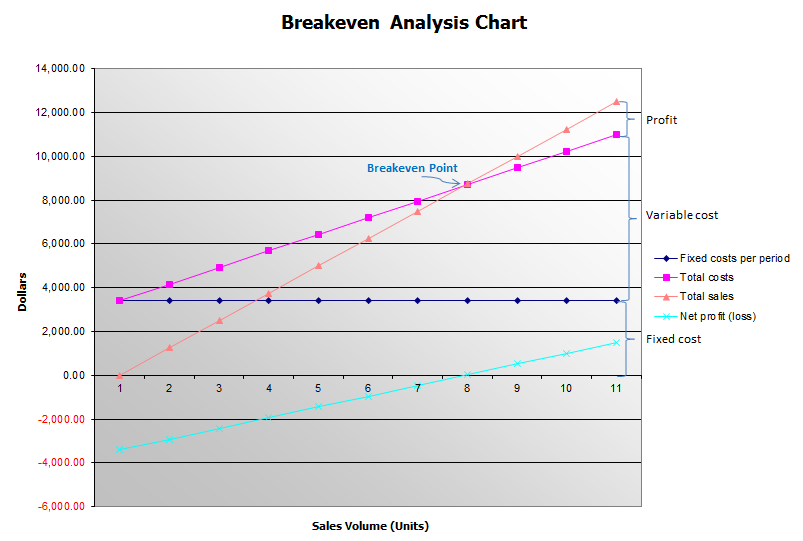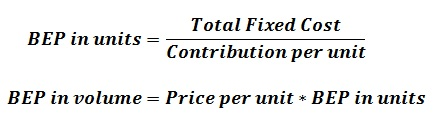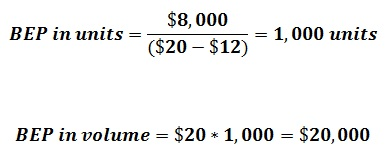# How to Calculate the Breakeven Point

As the breakeven point calculation is very essential for the business organizations in order to make strategic decisions, it is important to know how to calculate the breakeven point (BEP). The purpose of this article is to explain different methods used for calculating BEP.

## What is Breakeven Point (BEP)

The breakeven point refers to the point at which the total costs are equal to the total revenue generated. It is the point at which all the expenses are covered with the sales income. This point onwards the company generates profits.

## How to calculate breakeven point – Methods

There are usually two basic methods that can be used to calculate the Breakeven Point (BEP).

### Method 1: Using the BEP chart to calculate the Breakeven PointFixed costs: Costs that are fixed without any variations e.g. wages, rent and rates on business premises.

Variable costs: Costs that vary with the quantity of the products being produced or sold e.g. costs of materials produced.

Total cost: Fixed costs plus variable costs for any level of output.

Sales revenue: The price of the product multiplied by total sales.

Profit: The difference between total revenue and total cost (where the revenues are higher than costs).

Loss: The difference between total revenue and total cost (where costs are higher than the revenues).

### Method 2: Using BEP Formula to Calculate the Breakeven Point

Breakeven point (BEP) can also be calculated using a formula as stated below,For example:

If the price per unit is \$20, variable cost per unit is \$12, and total fixed cost is \$8,000, calculate the breakeven point.

When calculating the BEP using the Breakeven Point formula given above, answers can be derived as follows,## Why is it important to calculate the breakeven point?

Identifying this breakeven point is so crucial with regard to many reasons as stated below:

• In order to determine the remaining capacity after reaching the breakeven point. In other words, the maximum amount of profits that could be generated.
• Identify the impact towards profit if automation (fixed cost) is replaced in terms of human labour (variable cost).
• In a crisis, it helps the business to determine the losses.

In organizational perspective, managers are responsible for constantly monitoring the BEP, in order to reduce the BEP. There are several methods that can be used to do this.

• Cost analysis. It can be used to identify the costs that can be eliminated in order to reduce the BEP.
• Margin analysis. Closely monitor the product margins and work towards to increase sales of the highest-margin items, in order to reduce the BEP.
• Outsourcing. Most often focusing on outsourcing specific business functions and convert it into a variable cost per unit, which reduces the BEP.
• Pricing. Reduce or eliminate the special offers provided to the customers in order to increase the BEP.